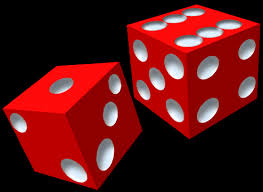# Dicey Probability

Probability Level 5Satvik and Agnishom are playing a game with $2$ standard dice. Both the dice are rolled together and the total is counted.

Satvik says that a total of $2$ will be rolled first.

Agnishom, whereas, says that two Consecutive totals of $7's$ will be rolled first.

They keep rolling the dice till one of them wins!

The probability that Satvik wins the game can be expressed as $\dfrac{a}{b}$ where $a$ and $b$ are co-prime positive integers.

Find the value of $a+b$.

×